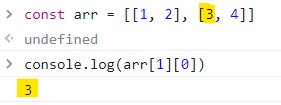# Nesting for loops cannot understand example code

Tell us what’s happening:
I tried my best to figure out how this code works out. So I just don’t understand, after first loop i = 1 (because of that i++)? But that’s not correct, because then arr[i][j] should show 1 4 instead of 1 2 3 4 5 6

``````  **Your code so far**
``````
``````
const arr = [
[1, 2], [3, 4], [5, 6]
];

for (let i = 0; i < arr.length; i++) {
for (let j = 0; j < arr[i].length; j++) {
console.log(arr[i][j]);
}
}
``````
``````  **Your browser information:**
``````

User Agent is: `Mozilla/5.0 (Windows NT 10.0; Win64; x64) AppleWebKit/537.36 (KHTML, like Gecko) Chrome/99.0.4844.74 Safari/537.36`

Challenge: Nesting For Loops

Looking at how `i` and `j` changes might give better idea what is happening - replace the current `console.log` call with: `console.log(i, j);`

2 Likes

Hi there!
`arr[i][j]` will output 1 item (integer 3), since we have a two-dimensional array.
This is how it works:
`arr` is the 2nd array, it’s `[3, 4]` and `arr` will output an element at `` index of `[3, 4]` array.So, `arr[i][j]` is the correct syntax to access an individual item in a two-dimensional array. `[i]` is accessing the inner array and `[j]` is accessing the item in the inner array.
Hope this helps!

1 Like

I tried that and console output is
0 0
0 1
1 0
1 1
2 0
2 1

The thing i don’t understand is how [j] becomes equal to 0 once again. isn’t j++; supposed to make it greater than what it already is?
And why [i] equals to 0 after [j] equals to 1, wasn’t [i] supposed to become 1 too?

A new j loop is being started on each iteration of the i loop. Try this code:

``````const arr = [
[1, 2], [3, 4], [5, 6]
];

for (let i = 0; i < arr.length; i++) {
console.log('\n* i loop, i =', i)
for (let j = 0; j < arr[i].length; j++) {
console.log('*** j loop, j =', j)
console.log('*** value = arr[' + i + '][' + j + ']', arr[i][j]);
}
}
``````
1 Like

This is what nesting does, for each iteration of the outer loop, the inner one loops until the breaking condition is met.

``````for (let i = 0; i < arr.length; i++) {
for (let j = 0; j < arr[i].length; j++) {
console.log(i, j);
}
}
``````

i.e. Outer loop - `i == 0`, inner loop will run from `j == 0` to `j < arr[i].length`. After that outer loop goes to the next iteration, with `i == 1`, and inner loop, again runs from start to the end.

1 Like

So basically once inner loop reaches its’ breaking point, only after that outer loop begins

Now i think i understand, thanks!

I would say that the outer loop starts an iteration where the inner loop does a complete set of iterations, the the outer loop does its next iteration where the inner loop does a complete set of iterations, the outer loop does is next iteraion.

It’s like a clock, 12 hours, 60 minutes each hour.

``````for (let hour = 0; hour < 12; hour++) {
for (let min = 0; min < 60; min++) {
waitSixtySeconds()
}
}
``````

For each hour, 60 minutes will tick by and the minutes will restart on each hour, for each iteration of the outer loop.

1 Like

This topic was automatically closed 182 days after the last reply. New replies are no longer allowed.